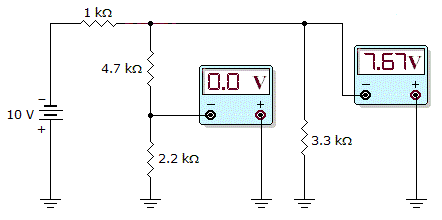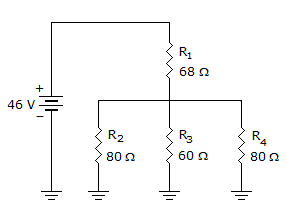# Electronics - Series-Parallel Circuits

### Exercise :: Series-Parallel Circuits - General Questions

21.Which circuit fault do the meter readings in the given figure indicate?

 A. The 1 kresistor is open. B. The 4.7 kresistor is open. C. The 2.2 kresistor is open. D. The 3.3 kresistor is open.

Explanation:

No answer description available for this question. Let us discuss.

22.How much current is flowing through R1 in the given circuit?

 A. 0.3 A B. 0.15 A C. 0.5 A D. 0.68 A

Explanation:

No answer description available for this question. Let us discuss.

23.

If R1 is in series with parallel connected to R2 and R3, what happens to total current if R2 opens?

 A. increases B. decreases C. remains the same D. cannot tell

Explanation:

No answer description available for this question. Let us discuss.

24.

The first goal(s) to accomplish in analyzing a complex series–parallel circuit is to:

 A. equate all parallel components B. equate all series components C. solve for all the voltage drops D. solve for the total current and resistance

Explanation:

No answer description available for this question. Let us discuss.

25.

If a series–parallel circuit has all 30-ohm resistors, what is the total resistance when R1 is in series with a parallel circuit consisting of R2 and R3?

 A. 10 ohms B. 20 ohms C. 45 ohms D. 90 ohms